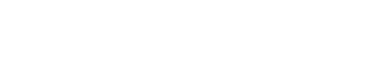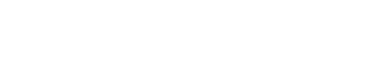In many applications, especially in test and measurement, you need to set the output voltage of an inverting buck/boost regulator with an external device or a digital-to-analog converter. In a conventional buck topology, this is simple: just direct current into the feedback node with a voltage supply with a series resistor, a current source, or a DAC, as shown in Figure 1.

In many applications, especially in test and measurement, you need to set the output voltage of an inverting buck/boost regulator with an external device or a digital-to-analog converter. In a conventional buck topology, this is simple: just direct current into the feedback node with a voltage supply with a series resistor, a current source, or a DAC, as shown in Figure 1.Figure 1. Programmable Voltage Using Buck Topology

However, if you need to change the voltage of a resistor in a buck-boost topology, it’s a bit of a hassle.

You can configure a buck regulator in a buck-boost topology by inverting the ground and VOUT potentials and placing the IC’s reference at the -VOUT potential. That is, the ground pin of the regulator IC is at -VOUT. Since the FB pin of the regulator is at -VOUT potential rather than ground potential, directing current into the FB pin is a little trickier. To direct current into the FB pin in an inverting buck-boost topology, you need to level shift the signal from the voltage source/DAC. This article will introduce you to some of the different methods.

Take the LMZM33606 as an example. The LMZM33606 is a step-down power module with a rated input voltage of 36V and a maximum load of 6A. Figure 2 illustrates how to set up the LMZM33606 as an inverting buck-boost regulator.Figure 2. Inverting Buck-Boost Using the LMZM33606

Method 1; Use a PNP level shifter

When using the LMZM33606 in these buck-boost applications, a programmable output voltage range of -15V to -5V can be achieved. With the current source method, you can turn down the output of the regulator by orders of magnitude. This allows the design’s default output to be -15V when setting the feedback divider resistors. When adding an external current source, you can set the regulator output to -5V. When the default output is -15V, the calculated high and low feedback values ​​are:

RFBT = 100k
RFBB = 7.42k.

An easy way to level-shift the ground-referenced signal to direct current into the FB pin is to use a single PNP-type bipolar transistor (BJT). Figure 3 illustrates how a single PNP can be used as a level shifter.

Figure 3. Deployment using a single PNP

The base of PNP Q1 is grounded and the reflector is connected to the DAC/voltage source through a resistor. When the voltage source is higher than the PNP base emission pull-down (VBE), the current described in Equation 1 is generated:Rext is set to 50kΩ. The FB node can apply Kirchhoff’s current law and you can calculate the current IX using Equation 2:Substituting Equation 1 into Equation 2 results in Equation 3, from which the programming voltage VX required to adjust the output voltage VOUT can be calculated:Converting Equation 3 to Equation 4 yields the VOUT that changes according to the value of VX:Equation 4 illustrates the dependence of VOUT on transistor VBE. Transistor VBE itself depends on the collector current, which, when varied with temperature, affects the degree to which VOUT is programmed.

The next method shows how to remove VBE from the equation. Figure 4 shows a circuit with two PNP transistors connected in a way that cancels the effects of VBE.

Method 2: Level shifter using two PNPs

Figure 4. Deployment of VBE offset with two PNPs

This method requires the use of two PNPs, preferably two PNP BJTs packaged in combination, to ensure a good match between the two transistors. The method can also reduce errors in output voltage programming.

The base of the Q1 transistor is connected to a programmable voltage source. The emitter is connected to the other positive rail via a series resistor RS, and the collector is connected to ground. This creates a VX VBE voltage at the transistor’s emitter. The emitter of Q2 transistor is connected to the emitter of Q1 through resistor RX. RX sets the current into the FB node. With the base grounded, the Q2 emitter node produces a VBE. Equation 5 calculates the current flowing to the emitter (ideal case):As explained earlier, the VBE of a transistor depends on the collector current, as described in Equation 6:Where IC is the collector current, IS is the saturation current, and VT is the thermal voltage.

If the collector currents of the two transistors are very different, the VBEs will not completely cancel each other out. Equation 7 illustrates the difference between the two VBEs of a transistor:Simplifies to Equation 8:where X is the ratio of the two collector currents.

If both collector currents are the same, VBE will cancel out completely. In the configuration shown in Figure 4, the value of RS needs to be set to ensure that the difference between the collector currents is not too large. In this example, the selected RS is 10k and the RX is 50k. The increase in VBE also varies with VT, which happens with temperature.

Method 3: Modified Wilson Current Mirror

Using a current mirror to match the collector current is a very efficient method. For this, the Wilson current mirror is a better choice than the conventional current mirror. Figure 5 is the schematic used in the Wilson current mirror.

Figure 5. Deployment with Wilson Current Mirror

In this method, there is another BJT with the base connected to the collector of Q1. The emitter of Q3 is connected to the VBE junction of the current mirror. The programmed current flows to the FB pin through the collector of the Q3 transistor.

Ignoring resistor RB for now, Equation 9 calculates the reference current imported into this setup as follows:Equation 10 gives the ratio of the lead-in current to the reference current.

At larger values ​​of transistor increments, it can be seen that the Wilson current mirror is much higher than the standard current mirror.

Wilson current mirrors do not completely eliminate the dependence on VBE. But there is an easy way to get around it. Connecting resistor RB from source VX to the base of the current mirror, as shown in Figure 5, forms a current that is added to reference current IX. Rewrite Equation 9 as Equation 11:

Note that the current introduced into the FB node is only based on the programmed voltage and is not affected by VBE.

Either method is used to create a programmable output voltage for the inverting rail with a few components. The complexity of the circuit will vary depending on the specific system requirements. For applications that require very high fidelity, the Wilson current mirror is the best solution because it produces a response close to the programmed voltage.

The Links:   NL6448AC33-18 CM25MD-24H GET PARTS